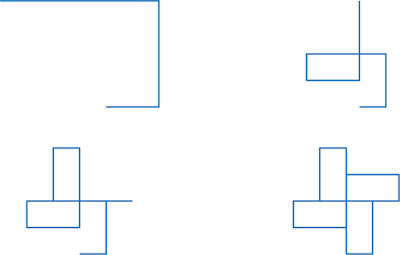## Monday, 23 October 2017

### Loop de loop patterns

During The Man Who Knew Infinity, Thomas produced a picture for Liz based on a random set of numbers taken from Donald Duck in Mathemagic Land.

The picture was a Loop de Loop pattern.

The idea is that you consider a moving pointer, known as a "turtle". The turtle is given instructions to move around and the picture is made up of the turtle's track history. The set of rules that the turtle follows is:
• the turtle moves along in a straight line a given distance. Here, the random numbers where used as the distances;
• after moving each distance the turtle is turned 90 degrees to the right;
• iterate the pattern until you get back to the beginning, or until you want to stop.
There are many websites that will allow you to produce such a picture using a programming language known as Logo.

For a simple example consider the list of numbers {50, 100, 150}. A single iteration of the Loop de Loop algorithm is given by

fd 50 rt 90 fd 100 rt 90 fd 150,

where "fd" means "go forward" the given amount and "rt" means "turn right" the given number of degrees. Following this set of instruction would produce a "J" type pattern, as shown below. Iterating the pattern can be done simply through

repeat 4[fd 50 rt 90 fd 100 rt 90 fd 150 rt 90].

This code will repeat the instructions 4 times and produce a "pin wheel" type pattern, shown below.Iterations of the code using the set of numbers {50, 100, 150}. Top left - one iteration, top right - two iterations, bottom left - three iterations, bottom right - four iterations.
The numbers Thomas used (although apparently not the numbers that Liz gave) were

{7, 9, 4, 8, 5, 7, 4, 3, 9, 2, 5, 7, 3, 8, 4, 9, 7, 5, 2, 6, 3}.

Four iterations take you back to the beginning point; the four iterations are illustrated below. Once, you have made your pattern, you can colour it in and make it funky.The four iterations of the Loop de Loop pattern, using the Donald Duck random number list.
Thomas generated his pictures using MatLab. The code is given below.

Why not produce your own Loop de Loop pattern and tweet it to us @PodcastMathsAt?

%%%%%%%%%%%%%%%%%%%%%%%%%%%%%%%%%%%%%%%%%%%%%%%%

% Clear all information
clear
close all
clc
N=[7 9 4 8 5 7 4 3 9 2 5 7 3 8 4 9 7 5 2 6 3]; %The list of numbers.

figure('position',[0 0 1 1]) %Set the figure space to fill the screen.

% Iteration 1
M=N;
theta=0;
x(1)=0;

for i=1:length(M)

x(i+1)=x(i)+M(i)*exp(theta*1i);
theta=theta+pi/2;
end
subplot(2,2,1)
plot(real(x),imag(x))
axis equal
axis off

% Iteration 2
M=[N N]
theta=0;
x(1)=0;
for i=1:length(M)

x(i+1)=x(i)+M(i)*exp(theta*1i);
theta=theta+pi/2;
end
subplot(2,2,2)
plot(real(x),imag(x))
axis equal
axis off

% Iteration 3
M=[N N N];
theta=0;
x(1)=0;

for i=1:length(M)

x(i+1)=x(i)+M(i)*exp(theta*1i);
theta=theta+pi/2;
end
subplot(2,2,3)
plot(real(x),imag(x))
axis equal
axis off

% Iteration 4
M=[N N N N];
theta=0;
x(1)=0;

for i=1:length(M)

x(i+1)=x(i)+M(i)*exp(theta*1i);
theta=theta+pi/2;
end
subplot(2,2,4)
plot(real(x),imag(x))
axis equal
axis off